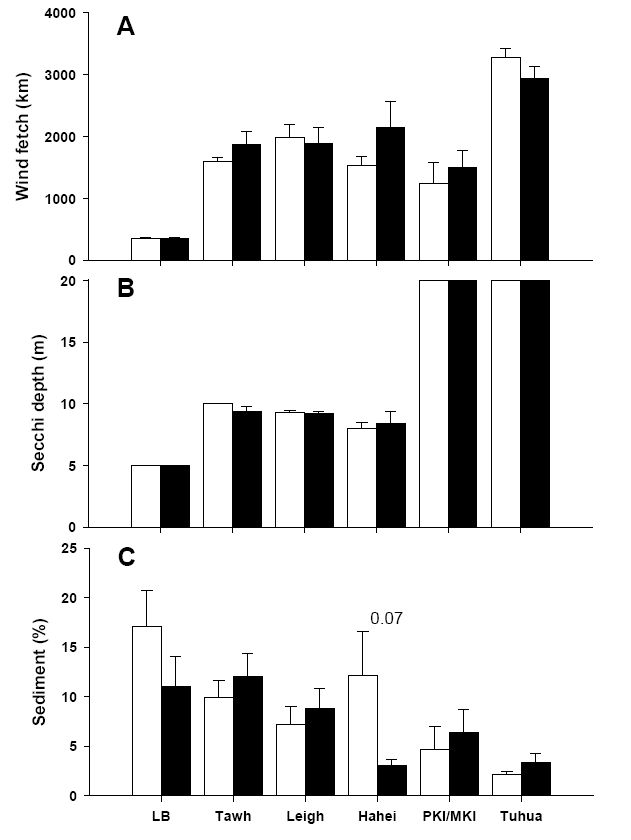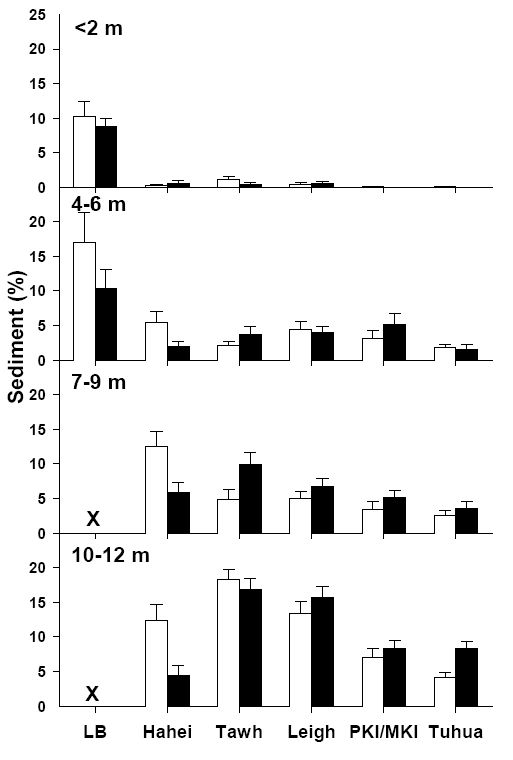### Nick T. Shears, Russell C. Babcock, and Anne K. Salomon. 2008. Context-dependent effects of fishing: Variation in trophic cascades across environmental gradients. Ecological Applications 18:1860–1873.

Appendix B. Analysis of environmental variation among locations, and between reserve and fished sites.

TABLE B1. Results from mixed model analysis of each environmental variable at reserve and fished sites. Variation in environmental variables: (a) across Location and Status, and (b) for Status within each location. Models were back-fitted by removing nonsignificant interaction terms. There was no variation in water clarity measurements among sites at Long Bay, PKI/MKI, and Tuhua so these could not be tested (indicated by “X”). Similarly, as single measurements in water clarity and wave exposure were made at each site we could not test for site-level effects.

 Variable Location Status Location × Status Site(Location × Status) (a) Sediment F5,65 = 5.49*** F1,65 = =0.43 - 0.118*** Water clarity F5,64 = 1022.32*** F1,65 = =0.05 - X Wave exposure F5,64 = 17.92*** F1,65 = =0.79 - X (b) Sediment Status Site(Status) Long Bay F1,9 = 1.11 P = 0.3199 0.297 P = 0.110 Tawharanui F1,8 = 0.43 P = 0.530 0.040 P = 0.327 Leigh F1,12 = 0.09 P = 0.772 0.299 P = 0.044 Hahei F1,8 = 4.25 P = 0.073 0.695 P = 0.054 PKIMKI F1,16 = 0.45 P = 0.514 1.870 P = 0.011 Tuhua F1,6 = 1.43 P = 0.276 0.1379 P = 0.148 Water clarity Status Site(Status) Long Bay X X Tawharanui F1,8 = 2.25 P = 0.172 X Leigh F1,13 = 0.02 P = 0.887 X Hahei F1,8 = 0.14 P = 0.720 X PKIMKI X X Tuhua X X Wave exposure Status Site(Status) Long Bay F1,8 = 0.01 P = .923 X Tawharanui F1,8 = 1.49 P = 0.256 X Leigh F1,13 = 0.07 P = 0.795 X Hahei F1,8 = 1.83 P = 0.213 X PKIMKI F1,16 = 0.39 P = 0.541 X Tuhua F1,6 = 2.02 P = 0.205 XFIG. B1. Environmental variation among locations and between reserve (open bars) and fished (shaded bars) sites: Wave exposure (A), water clarity (B) and sediment (C). Note: secchi depth was >20 m at all offshore sites, but could not be measured accurately beyond 20 m depth. No significant differences in any of the environmental variables were found between reserve and fished sites.FIG. B2. Depth related variation in sediment cover (% + SE) among locations and between reserve (open bars) and fished (shaded bars) sites.

[Back to A018-065]